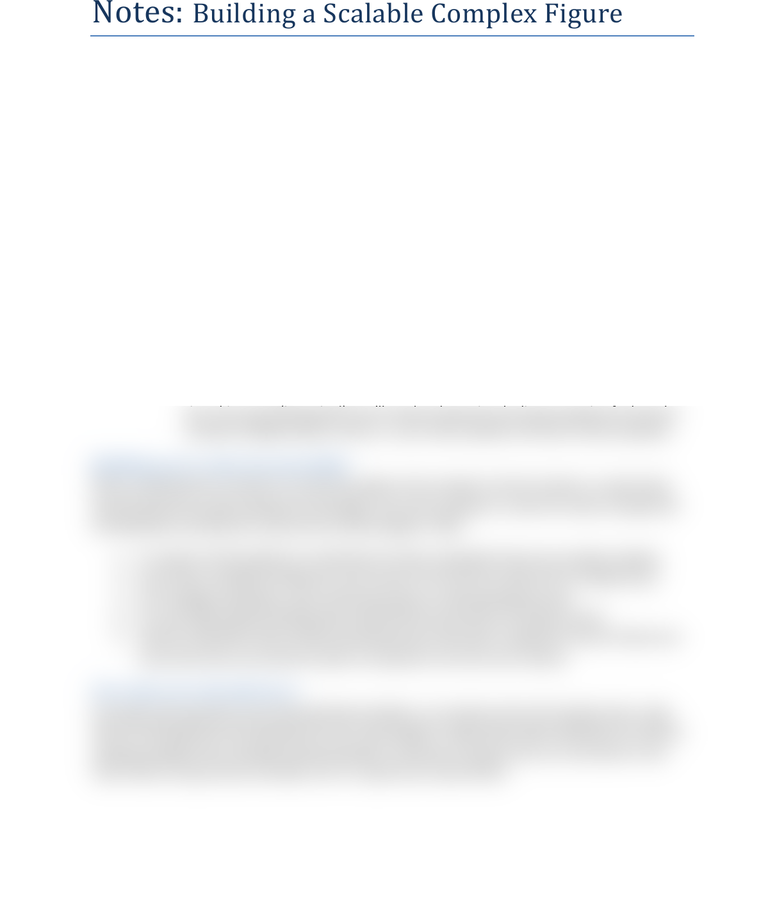# CSCI 2170 Lecture 3: Building a Complex Figure

10 views1 pages
School
Course
ProfessorAP CS Name: ________________________
Notes: Building a Scalable Complex Figure
Recommendations for managing complexity:
1 Design the program by thinking about the steps or methods needed.
Write an English description (psuedocode!) of steps required.
Use this description to decide the methods and loops needed.
2 Determine the nested loops. (Note: These are just guidelines for figures; actual use may vary!)
Outer loop (top) used to determine the number of lines (aka rows)
Remember to have a new line (i.e., println()) at the end of the outer loop!
If a characters number does not vary (i.e., only appears once), then it simply needs to be
printed (using the print() method)
If a characters number varies per line, then you need a for loop and require a formula
3 Create a table that counts out the patterns of the characters in relation to the line they are on. Use
this table to find an equation (e.g., y = mx + b, the linear equation utilizing slope and y-intercept).
Use a for loop, starting at 1, going to the desired length. Desired length is determined by:
For each set of characters, use table to determine the pattern and then the linear equation.
In general (but not always):
Pattern increases: constant in the linear equation does not change based on scaling size
Pattern decreases: constant in linear equation typically changes based on the scaling
size. This means (inception!), youll need to determine the linear equation for how the
constant changes based on size (i.e., your linear equation will have a linear equation).
Write a small portion of code for to start the program. (For example: the first method, a couple loops
that build the first couple characters of the figure, etc.) Then compile it, check the results, and get that
working before writing more code and the whole program. Why?
1. It is easier to find problems in small pieces of code, especially if there are multiple mistakes.
2. If you have a repeated mistake you can correct it once and not need to fix it multiple times.
3. This manages complexity, starts small and builds up, learning along the way.
4. You can copy & paste working code to add further occurrences, but keep in mind:
5. Look for redundant code to better decompose your final code. In general: If lines of code occur
more than twice, you should consider turning them into their own method.
If you get stuck, go back to your lab worksheet problems, our previous lectures & sample code, using
them as starting points and examples for your new program. A good way to get unblocked is to start by
editing a program that is already working. Qualifier: Eventually, the goal is for you to be able to write
code without using previous examples, but it’s a good way to get started.
Unlock document

This preview shows half of the first page of the document.
Unlock all 1 pages and 3 million more documents.